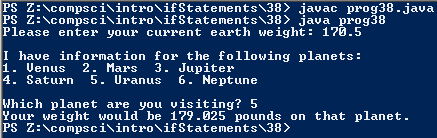# Assignemnt #38, Space Boxing

## Code

```      /// Name: Ales Pinder
/// Period: 5
/// Program Name: Space Boxing
/// File Name: prog38.java
/// Date Complete: 10/29/15

import java.util.Scanner;

public class prog38
{
public static void main( String[] args )
{
Scanner keyboard = new Scanner (System.in);

double weight;
weight = keyboard.nextDouble();

System.out.println( "\nI have information for the following planets: \n1. Venus  2. Mars  3. Jupiter  \n4. Saturn  5. Uranus  6. Neptune" );

System.out.print( "\nWhich planet are you visiting? " );

if ( answer == 1 )
{
System.out.println(  "Your weight would be " + ( weight * 0.78 ) + " pounds on that planet." );
}
else if ( answer == 2 )
{
System.out.println( "Your weight would be " + ( weight * 0.39 ) + " pounds on that planet." );
}
else
{
if ( answer == 3 )
{
System.out.println( "Your weight would be " + ( weight * 2.65 ) + " pounds on that planet." );
}
else if ( answer == 4 )
{
System.out.println( "Your weight would be " + ( weight * 1.17 ) + " pounds on that planet." );
}
else
{
if ( answer == 5 )
{
System.out.println( "Your weight would be " + ( weight * 1.05 ) + " pounds on that planet." );
}
else if ( answer == 6 )
{
System.out.println( "Your weight would be " + ( weight * 1.23 ) + " pounds on that planet." );
}
else
{
if ( answer < 1 )
{
System.out.println( "That is not a planet!" );
}
else if ( answer < 6 )
{
System.out.println( "That is not a planet!" );
}
}
}
}
}
}
```

### Picture of the output